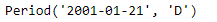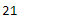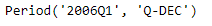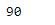# Python | Pandas Period.dayofyear

• Last Updated : 06 Jan, 2019

Python is a great language for doing data analysis, primarily because of the fantastic ecosystem of data-centric python packages. Pandas is one of those packages and makes importing and analyzing data much easier.

Pandas` Period.dayofyear` attribute returns the day of the year for the given Period object. It returns an integer value.

Attention geek! Strengthen your foundations with the Python Programming Foundation Course and learn the basics.

To begin with, your interview preparations Enhance your Data Structures concepts with the Python DS Course. And to begin with your Machine Learning Journey, join the Machine Learning - Basic Level Course

Syntax : Period.dayofyear

Parameters : None

Return : day of the year

Example #1: Use `Period.dayofyear` attribute to find the day of the year in the given Period object.

 `# importing pandas as pd``import` `pandas as pd`` ` `# Create the Period object``prd ``=` `pd.Period(freq ``=``'D'``, year ``=` `2001``, month ``=` `1``, day ``=` `21``)`` ` `# Print the Period object``print``(prd)`

Output :Now we will use the `Period.dayofyear` attribute to find the day of the year in the given object.

 `# return the day value ``prd.dayofyear`

Output :As we can see in the output, the `Period.dayofyear` attribute has returned 21 indicating that the following date was the 21st day of the year.

Example #2: Use `Period.dayofyear` attribute to find the day of the year in the given Period object.

 `# importing pandas as pd``import` `pandas as pd`` ` `# Create the Period object``prd ``=` `pd.Period(freq ``=``'Q'``, year ``=` `2006``, quarter ``=` `1``)`` ` `# Print the object``print``(prd)`

Output :Now we will use the `Period.dayofyear` attribute to find the day of the year in the given object.

 `# return the day value ``prd.dayofyear`

Output :As we can see in the output, the `Period.dayofyear` attribute has returned 90 indicating that the following date was the 90th day of the year.

My Personal Notes arrow_drop_up